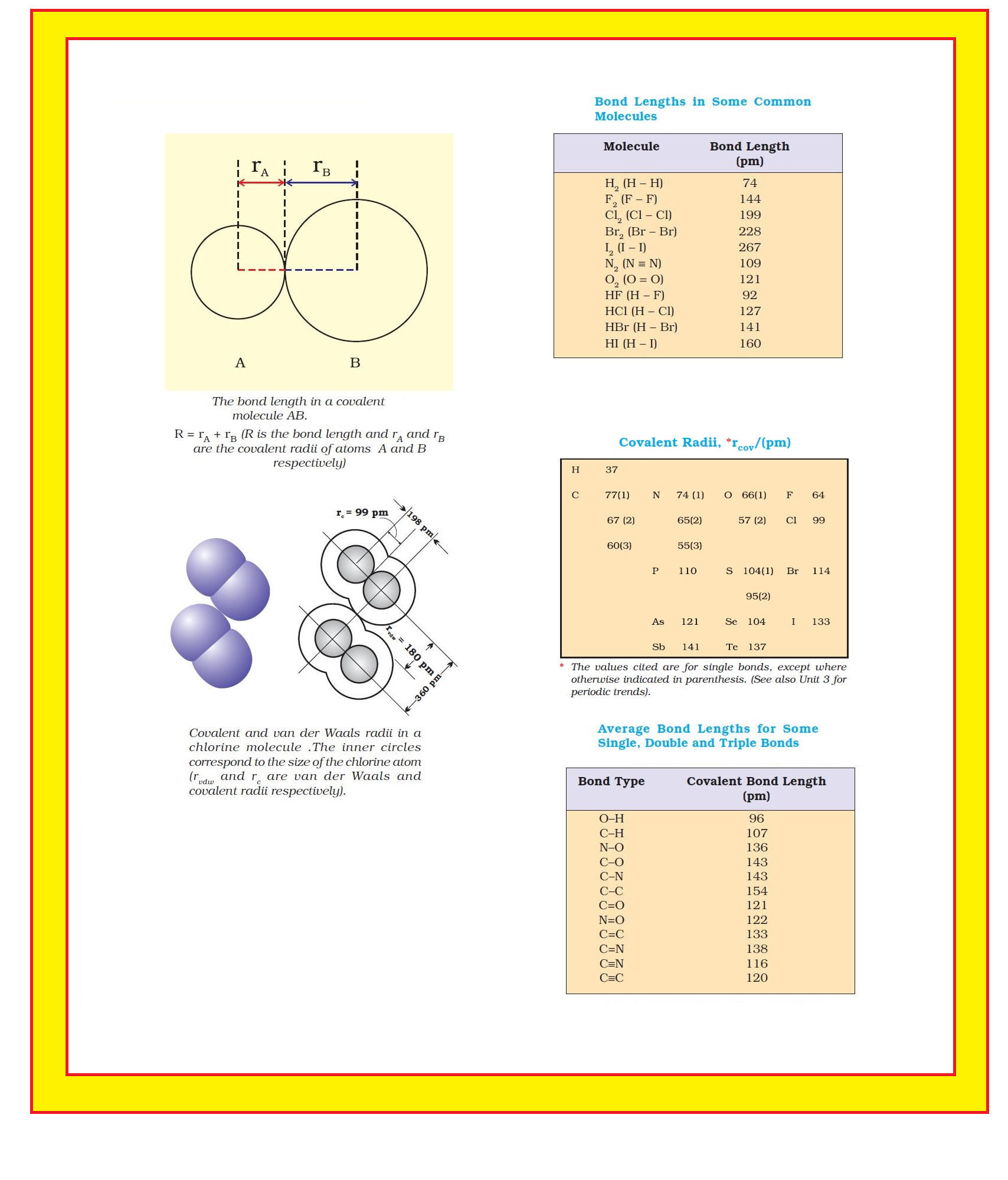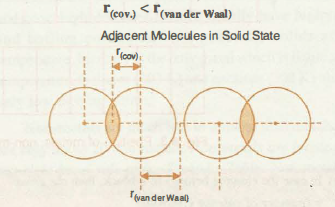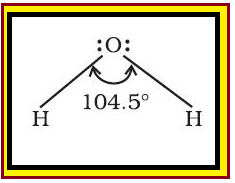Chemistry Bond Parameters-I
Click for Only Video

### Topics Covered :

● Bond Parameters
● Bond Length
● Bond Angle
● Bond Enthalpy

### Bond Parameters :

Bond parameter are given as follow :

### Bond Length :color{red}("Definition :") Bond length is defined as the equilibrium distance between the nuclei of two bonded atoms in a molecule.

color{green} ✍️ color{green} mathbf("KEY CONCEPT")
When atoms come closer to each other then attraction takes place between them and P.E. keeps on decreasing till at a particular distance and here potential energy is minimum. If the atoms are brought closer, the repulsion starts and therefore potential energy of the system begins to increase. Thus at equilibrium distance the atoms keep on vibrating about their mean positions.
● Bond lengths are measured by spectroscopic, X-ray diffraction and electron-diffraction techniques.

● Each atom of the bonded pair contributes to the bond length (Fig. 4.1).

● In the case of a covalent bond, the contribution from each atom is called the covalent radius of that atom.

color{green}("Covalent Radii "): ● The covalent radius is measured approximately as the radius of an atom’s core which is in contact with the core of an adjacent atom in a bonded situation.

● The covalent radius is half of the distance between two similar atoms joined by a covalent bond in the same molecule.

color{green}("van der Waal's Radii" ): ● The van der Waals radius represents the overall size of the atom which includes its valence shell in a non-bonded situation.

● The van der Waals radius is half of the distance between two similar atoms in separate molecules in a solid.

=> Covalent and van der Waals radii of chlorine are depicted in Fig.

=> Some typical average bond lengths for single, double and triple bonds are shown in Table.

=> Bond lengths for some common molecules are given in Table

=> The covalent radii of some common elements are listed in Table
•color{red}("Factors affecting bond length ") :
=> BOND LENGTH prop SIZE OF ATOMS
Eg. HI>HBr>HCl.
=> BOND LENGTH prop 1/text(MULTIPLICITY)

### Why is r_text(van der Waal) > r_text(cov.) in Covalent Compounds?=> The van der Waals radius is greater than the covalent radius because it does not take into account overlap.

=> The overlap that exists between the two electron clouds is what causes the covalent radius to be smaller than the van der Waals radius.
• Factors affecting bond length:
=> size of the atoms:The bond length increases with increase in size of atoms. For eg. HI > HBr > HC l > H F.
=> Multiplicity of bond:The bond length decreases with multiplicity of the bond. C equiv C < C = C < C - C.

### Bond Angle :color{red}("Definition :") It is defined as the angle between the orbitals containing bonding electron pairs around the central atom in a molecule/complex ion.

● Bond angle is expressed in degree which can be experimentally determined by spectroscopic methods.

● It gives some idea regarding the distribution of orbitals around the central atom in a molecule/complex ion and hence it helps us in determining its shape.

color{red}("Example ") H–O–H bond angle in water can be represented as shown in fig.

=> color{red}("Factors affecting bond angle ") :
•Hybridisation: Bond angle is directly proportional to % of s character.
•Lone pair: Bond angle is inversely proportional to number of lone pair.
•Central atom: Bond angle is directly proportional to electronegativity of central atom.

### Bond Enthalpy :

color{red}("Definition :") It is defined as the amount of energy required to break one mole of bonds of a particular type between two atoms in a gaseous state.

● The unit of bond enthalpy is kJ mol^(–1).

color{red}("Example :") The H – H bond enthalpy in hydrogen molecule is 435.8 kJ mol^(–1).

H_2 (g) → H (g) + H (g) ; Delta_a H^v = 435.8 kJ mol^(-1)

Similarly, the bond enthalpy for molecules containing multiple bonds, for example O_2 and N_2 will be as under :

O_2 (O = O) (g) → O(g) +O(g) ; Delta_a H^v = 498 kJ mol^(-1)

N_2 (N = N ) (g) → N (g) +N(g) ; Delta_a H^v = 946.0 kJ mol^(-1)

=> It is important that larger the bond dissociation enthalpy, stronger will be the bond in the molecule.

=> For a heteronuclear diatomic molecules like HCl, we have

HCl (g) → H (g) +Cl (g) ; Delta_a H^v = 431.0 kJ mol^(-1)

=> In case of polyatomic molecules, the measurement of bond strength is more complicated.

color{red}("Example ") In case of H_2O molecule, the enthalpy needed to break the two O – H bonds is not the same.

H_2O(g) → H(g) + OH (g) ; Delta_a H_1^v = 502 kJ mol^(-1)

OH(g) → H (g) +O(g) ; Delta_a H_2^v = 427 kJ mol^(-1)

● The difference in the Δ_aH^v value shows that the second O – H bond undergoes some change because of changed chemical environment.

● This is the reason for some difference in energy of the same O – H bond in different molecules like C_2H_5OH (ethanol) and water.

● Therefore in polyatomic molecules, the term mean or average bond enthalpy is used.

● It is obtained by dividing total bond dissociation enthalpy by the number of bonds broken as explained below in case of water molecule,

color{green}("Average bond enthalpy") = (502+427)/2

 = 464.5 kJ mol^(-1)

• color{red}("Factors affecting bond enthalpy ") :
=> Greater the size of atoms, greater is the bond length and less is bond dissociation enthalpy.
=> For the bond between the same two atoms,greater is multiplicity of bond,greater is the bond dissociation enthalpy. Eg. H-H => Greater the number of lone pairs of electrons present on the bonded atoms, greater is the repulsion between the atoms and hence less is BDE.